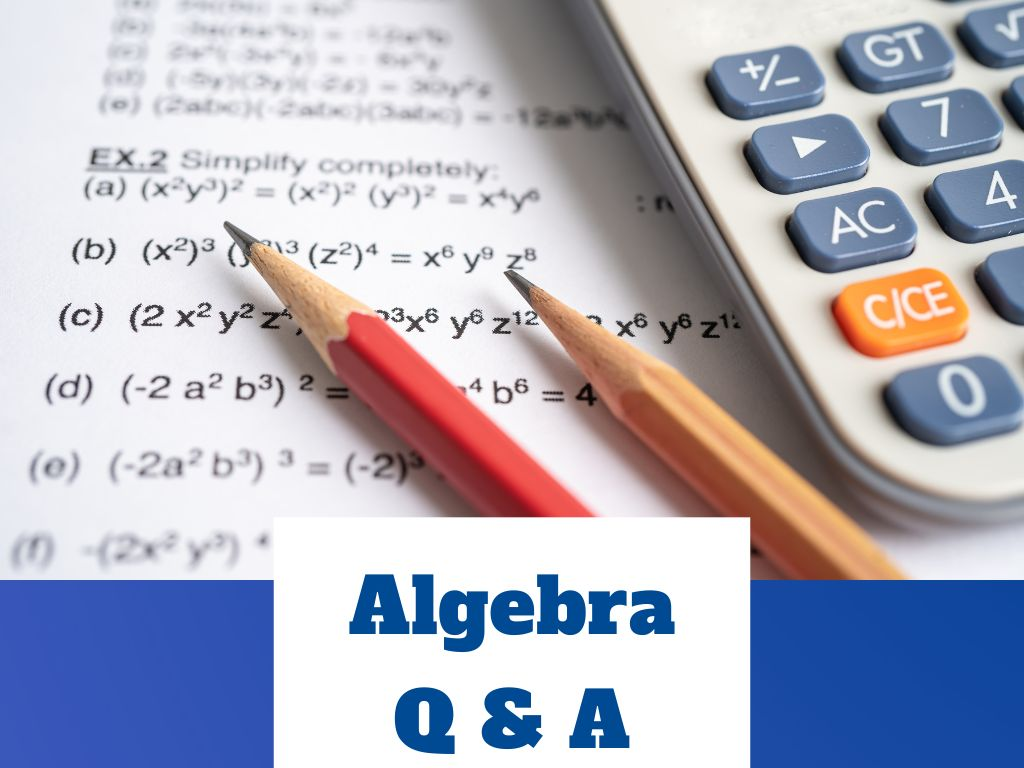# Algebra Q & A

Q 1) Are polynomials linear?

Ans. – Yes, polynomials are linear and called linear polynomials. The standard form of a linear polynomial is ax + b where a ≠ 0. Example – 2x + 3, x – 5, 7x, etc.

Q 2) Are polynomials closed under division?

Ans. – No, polynomials are not closed under division because, in the division of two polynomials, it is not necessary to get the polynomial each time as a result. Example – If we divide x2 by x then we get x but if we divide in reverse order means x by x2 then we get 1/x or x-1.

x2 / x = x (polynomial) but x / x2 = 1/x or x-1 (not polynomial)

Q 3) Are polynomials closed under addition?

Ans. – Yes, polynomials are closed under addition because when we add two or more polynomials, we get a polynomial, as a result, every time. Example – (1) 8x5 + 7x5 = 15x5 (2) 2x + 3x2 = 3x2 + 2x

Q 4) Are polynomials closed under subtraction?

Ans. – Yes, polynomials are closed under subtraction because when we subtract a polynomial from another polynomial, we get a polynomial, as a result, every time. Example – (1) 7x3 – 3x3 = 4x3 (2) 6x – 3x = 3x

Q 5) Are polynomials closed under multiplication?

Ans. – Yes, polynomials are closed under multiplication because when we multiply two or more polynomials, we get a polynomial, as a result, every time. Example – (1) x2 × 2x2 = 2x4 (2) 2x × 3x = 6x2

Q 6) Can polynomials have negative exponents?

Ans. – No, polynomials cannot have negative exponents because polynomials can have only positive exponents or non-negative integers.

Q 7) Can polynomials have square roots?

Ans. – No, polynomials can not have variables in square roots because of the definition of polynomials.

Q 8) Can polynomials have fractions?

Ans. – No, polynomials cannot have variables in the form of fractions.

Q 9) Can polynomials have radicals?

Ans. ­– According to the rules of polynomials, polynomials cannot have radicals.

Q 10) Can polynomials have asymptotes?

Ans. – Yes, polynomials having degree 0 (horizontal asymptotes) and degree 1 (oblique asymptotes) have asymptotes because their graphs are straight lines.

Q 11) Can polynomials have variable exponents?

Ans. – No, polynomials cannot have variable exponents because variable exponents are present in the exponential function.

Q 12) How polynomials are used in everyday life?

Ans. – Polynomials are used in the field of mathematics and science. In everyday life, polynomials are used in the field of construction as modeling of buildings and objects and used in industries. Polynomials are also used in the field of marketing, finance, stocks, etc.

Q 13) Which polynomials are prime?

Ans. – A polynomial that cannot be factored into a lower-degree polynomial is called a prime polynomial or an irreducible polynomial. Example – 2x, x2 + x + 1, x2 – 2, etc.

Q 14) What polynomials cannot be factored?

Ans. – Polynomials that are prime or irreducible cannot be factored.

Q 15) What is the standard form of polynomial x2 + 3x + 2?

Ans. – The standard form of the polynomial x2 + 3x + 2 is ax2 + bx + c.

Algebra Q & A in Hindi

1.